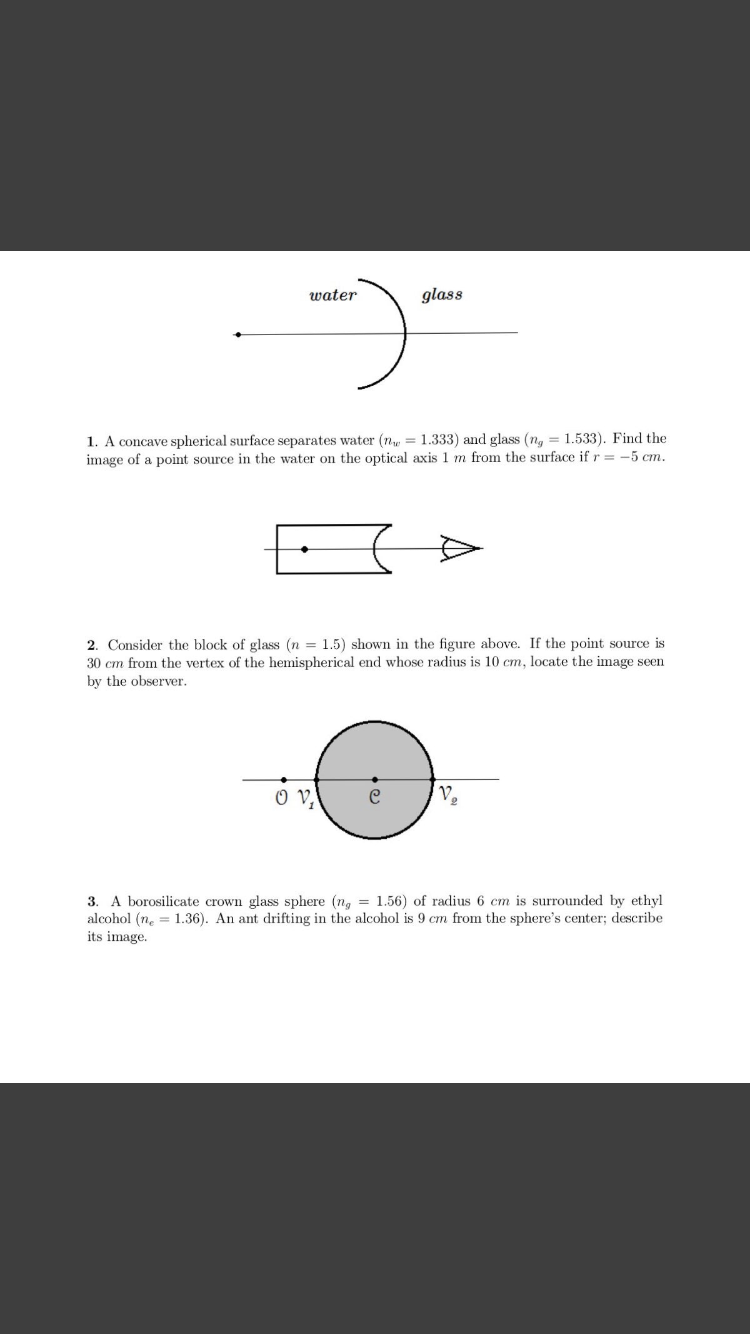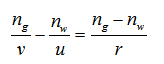# glasswater1. A concave spherical surface separates water (n = 1.333) and glass (ng = 1.533). Find theimage of a point source in the water on the optical axis 1 m from the surface if r = -5 cm.2. Consider the block of glass (n = 1.5) shown in the figure above. If the point source is30 cm from the vertex of the hemispherical end whose radius is 10 cm, locate the image seenby the observer.O V,A borosilicate crown glass sphere (n, = 1.56) of radius 6 cm is surrounded by ethylalcohol (n, = 1.36). An ant drifting in the alcohol is 9 cm from the sphere's center; describeits image.3.

Question
29 viewshelp_outlineImage Transcriptioncloseglass water 1. A concave spherical surface separates water (n = 1.333) and glass (ng = 1.533). Find the image of a point source in the water on the optical axis 1 m from the surface if r = -5 cm. 2. Consider the block of glass (n = 1.5) shown in the figure above. If the point source is 30 cm from the vertex of the hemispherical end whose radius is 10 cm, locate the image seen by the observer. O V, A borosilicate crown glass sphere (n, = 1.56) of radius 6 cm is surrounded by ethyl alcohol (n, = 1.36). An ant drifting in the alcohol is 9 cm from the sphere's center; describe its image. 3. fullscreen
check_circle

Using the expression for refraction at concave surface,...

### Want to see the full answer?

See Solution

#### Want to see this answer and more?

Solutions are written by subject experts who are available 24/7. Questions are typically answered within 1 hour.*

See Solution
*Response times may vary by subject and question.
Tagged in